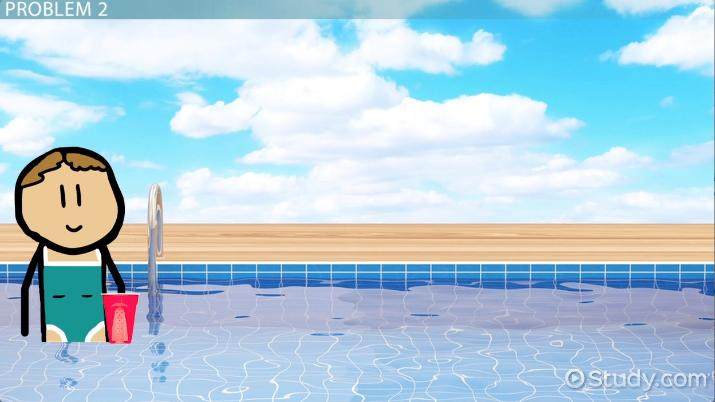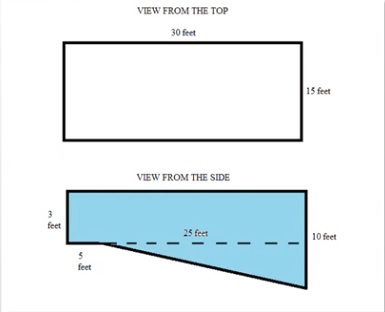# Volume: Real-World Geometry Problems

Lesson Transcript
Instructor: Elizabeth Foster

Elizabeth has been involved with tutoring since high school and has a B.A. in Classics.

Volume, the amount of space an object takes up, can be calculated using geometric principles applied to real-world situations. Understand the application of geometry on volume through a pair of practice problems. Updated: 11/15/2021

## Volume

In this lesson, we'll be talking about how you can use volume to solve problems in the real world. But first, we'll warm up with a very brief review of some volume formulas. In a 3-dimensional shape, volume describes the amount of space that the shape encloses. In other words, how big is the space inside the shape?

The volume of a cube is equal to the length of one side to the 3rd power. So for example, if you had a cubical box with edges 2 feet long, the volume would be 8 cubic feet.

The volume of a rectangular prism is equal to the length times the width times the height. Let's say your box isn't perfectly square. It's 2 feet long, 3 feet wide, and 4 feet high. In that case, the volume would be 2 * 3 * 4, or 24 cubic feet.

The volume of a cylinder is the area of the base times the height. An example of this is a soup can. If the radius of the soup can is 2 inches and the height is 4 inches, then the volume of the can is pi x 2^2 * 4, or roughly 50.24 cubic inches.

The same goes for a 3-D shape where one side is a triangle, like those boxes you get if you buy just one slice of pizza. Just take the area of the triangle base times the height.

If you're still fuzzy on those, you might want to review them in other lessons before you move on with this one because now we're going to see how we can apply them in real life.An error occurred trying to load this video.

Try refreshing the page, or contact customer support.

Coming up next: How to Calculate Simple Conditional Probabilities

### You're on a roll. Keep up the good work!

Replay
Your next lesson will play in 10 seconds
• 0:14 Volume
• 1:38 Problem 1
• 3:49 Problem 2
• 6:37 Lesson Summary
Save Save

Want to watch this again later?

Timeline
Autoplay
Autoplay
Speed Speed

## Problem 1

First off, let's fill up a backyard pool.

Anastasia pays 50 cents per cubic foot of water. She wants to fill her pool, which looks like this. The pool is 15 feet wide by 30 feet long. It's shaped like a rectangle, with a depth changing from 3 feet at the shallow end to 10 feet at the deep end, as shown in the picture. How much will Anastasia pay to fill the pool?You can see from the diagram that the pool is 3 feet deep for 5 of its length, and then steadily slopes downward to a depth of 10 feet over the next 25 feet of its length. We can break this pool up into two different shapes: there's a rectangular prism, above the dotted line, and then a triangular prism, below the dotted line. To take our example, it's basically a rectangular box on top of a pizza-slice box. To find the volume of the whole pool, we'll find the rectangular prism and then add it to the triangular prism.

We'll start with the rectangle. This rectangle is 3 feet deep, 15 feet wide, and 30 feet long. So its volume is 3 * 15 * 30, or 1,350 cubic feet.

Now we'll find the volume of the triangular prism. To do this, we'll take the area of the triangular base and multiply it by the distance between each base. In this case, the base is 7 * 25 / 2 feet squared, or 87.5 square feet. Multiply that by 15 to find the total volume of the prism: 1,312.5 cubic feet.

Now we add 1,350 + 1,312.5 to get 2,662.5 cubic feet for the volume of the entire pool. Anastasia pays 50 cents per cubic foot of water, so we'll have to multiply that by 0.50 to get the total price she pays: \$1,331.25.

## Problem 2

Now you have your pool filled, but if you want a pool party, you need some drinks! So let's head right into the second problem.

Anastasia has a lot of friends, so for Anastasia's pool party, Classic Catering has to deliver 500 gallons of soda, which is equivalent to 115,500 cubic inches of soda. The soda is all in cans with a height of 4 inches and a diameter of 4 inches. The soda company is delivering the cans in dollies with a packable area of 1 foot wide by 1 foot long by 3 feet high. How many dollies does Classic Catering need to deliver all the soda?

This sounds complicated, but let's break it down and take it one step at a time. First we'll figure out how many cans we're actually talking about.

To unlock this lesson you must be a Study.com Member.

## Practice:Volume: Real-World Geometry Problems Quiz

1/5 completed

### In 2005, Josh paid 25 cents per cubic foot of water. In 2015, a drought has made water more expensive, and now he pays 30 cents per cubic foot. Josh owns a swimming pool that measures 10 feet wide by 20 feet long by 8 feet deep. How much MORE does Josh pay to fill up his pool in 2015 than in 2005?

Create Your Account To Take This Quiz

As a member, you'll also get unlimited access to over 84,000 lessons in math, English, science, history, and more. Plus, get practice tests, quizzes, and personalized coaching to help you succeed.

It only takes a few minutes to setup and you can cancel any time.

### Register to view this lesson

Are you a student or a teacher?